Home | | Business Maths 12th Std | Methods of finding initial Basic Feasible Solutions

# Methods of finding initial Basic Feasible Solutions

Method:1 North-West Corner Rule (NWC), Method:2 Least Cost Method (LCM), Method:3 VogelŌĆÖs Approximation Method(VAM)

Methods of finding initial Basic Feasible Solutions

There are several methods available to obtain an initial basic feasible solution of a transportation problem. We discuss here only the following three. For finding the initial basic feasible solution total supply must be equal to total demand.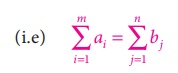## Method:1North-West Corner Rule (NWC)

It is a simple method to obtain an initial basic feasible solution. Various steps involved in this method are summarized below.

Step 1: Choose the cell in the north- west corner of the transportation Table10.1 and allocate as much as possible in this cell so that either the capacity of first row (supply)is exhausted or the destination requirement of the first column(demand) is exhausted. (i.e) x11 = min(a1,b1)

Step 2: If the demand is exhausted (b1 < a1), move one cell right horizontally tothe second column and allocate as much as possible.(i.e) x12= min (a1 ŌĆōx11, b2)

If the supply is exhausted (b1 > a1), move one cell down vertically to the second row and allocateas much as possible.(i.e)x21 = min (a2,b1 ŌĆō x11)

If both supply and demand are exhausted move one cell diagonally and allocate as much as possible.

Step 3: Continue the above procedure until all the allocations are made

Example 10.1

Obtain the initial solution for the following problemSolution:

Here total supply = 5+8+7+14=34, Total demand = 7+9+18=34

(i.e) Total supply =Total demandŌł┤The given problem is balanced transportation problem.

Ōł┤ we can findan initial basic feasible solution to the given problem.

From the above table we can choose the cell in the North West Corner. Here the cell is (1,A)

Allocate as much as possible in this cell so that either the capacity of first row is exhausted or the destination requirement of the first column is exhausted.

i.e. x11 = min (5,7) = 5Reduced transportation table isNow the cell in the North west corner is (2, A)

Allocate as much as possible in the first cell so that either the capacity of second row is exhausted or the destination requirement of the first column is exhausted.

i.e. x12 = min (2,8) = 2Reduced transportation table isHere north west corner cell is (2,B) Allocate as much as possible in the first cell so that either the capacity of second row is exhausted or the destination requirement of the second column is exhausted.

i.e. x22 = min (6,9) = 6Reduced transportation table is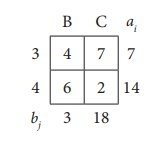Here north west corner cell is (3,B).

Allocate as much as possible in the first cell so that either the capacity of third row is exhausted or the destination requirement of the second column is exhausted.

i.e. x32 = min (7,3) = 3Reduced transportation table isHere north west corner cell is (3,C) Allocate as much as possible in the first cell so that either the capacity of third row is exhausted or the destination requirement of the third column is exhausted.

i.e. x33 = min (4,18) = 4Reduced transportation table and final allocation is x44 = 14Thus we have the following allocationsTransportation schedule : 1ŌåÆ A, 2ŌåÆA, 2ŌåÆB, 3ŌåÆB, 3ŌåÆC, 4ŌåÆC

The total transportation cost.

= (5 ├Ś 2) + (2 ├Ś 3) +(6 ├Ś 3)+(3 ├Ś 4)+(4 ├Ś 7) + (14 ├Ś 2)

= Rs.102

Example 10.2

Determine an initial basic feasible solution to the following transportation problem using North West corner rule.Here Oi and Dj represent ith origin and jth destination.

Solution:

Given transportation table is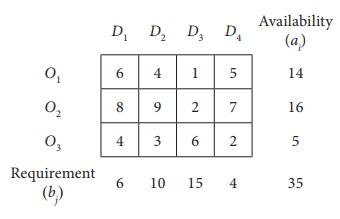Total Availability = Total Requirement

Ōł┤ The given problem is balanced transportation problem.

Hence there exists a feasible solution to the given problem.

First allocation: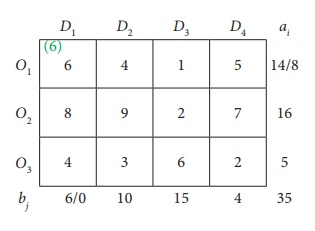Second allocation:Third Allocation:Fourth Allocation:Fifth allocation:Final allocation:Transportation schedule : O1ŌåÆ D1, O1ŌåÆD2, O2ŌåÆD2, O2ŌåÆD3, O3ŌåÆD3, O3ŌåÆD3.

The transportation cost

= (6 ├Ś 6)+(8 ├Ś 4)+(2 ├Ś 9)+(14 ├Ś 2)+(1 ├Ś 6)+(4 ├Ś 2) =Rs.128

## Method:2 Least Cost Method (LCM)

The least cost method is more economical than north-west corner rule,since it starts with a lower beginning cost. Various steps involved in this method are summarized as under.

Step 1: Find the cell with the least(minimum) cost in the transportation table.

Step 2: Allocate the maximum feasible quantity to this cell.

Step:3: Eliminate the row or column where an allocation is made.

Step:4: Repeat the above steps for the reduced transportation table until all the allocations are made.

Example 10.3

Obtain an initial basic feasible solution to the following transportation problem using least cost method.Here Oi and Dj denote ith origin and jth destination respectively.

Solution:

Total Supply = Total Demand = 24

Ōł┤ The given problem is a balanced transportation problem.

Hence there exists a feasible solution to the given problem.

Given Transportation Problem is:The least cost is 1 corresponds to the cells (O1, D1) and (O3, D4)

Take the Cell (O1, D1) arbitrarily.

Allocatemin (6,4) = 4 units to this cell.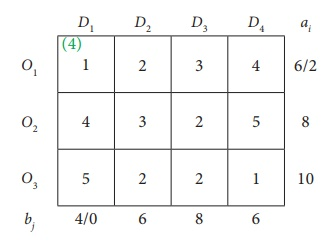The reduced table isThe least cost corresponds to the cell (O3, D4). Allocate min (10,6) = 6 units to this cell.The reduced table isThe least costis 2 corresponds to the cells (O1, D2), (O2, D3), (O3, D2), (O3, D3)

Allocate min (2,6) = 2 units to this cell.The reduced table is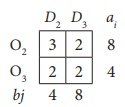The least cost is 2 corresponds to the cells (O2, D3), (O3, D2), (O3, D3)

Allocate min ( 8,8) = 8 units to this cell.The reduced table isHere allocate 4 units in the cell (O3, D2)Thus we have the following allocations:Transportation schedule :

O1ŌåÆ D1, O1ŌåÆD2, O2ŌåÆD3, O3ŌåÆD2, O3ŌåÆD4

Total transportation cost

=  (4├Ś1)+ (2├Ś2)+(8├Ś2)+(4├Ś2)+(6├Ś1)

=  4+4+16+8+6

=Rs. 38.

Example 10.4

Determine how much quantity should be stepped from factory to various destinations for the following transportation problem using the least cost method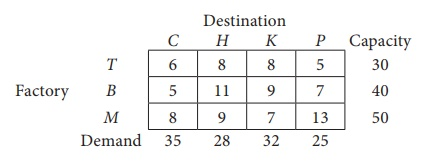Cost are expressed in terms of rupees per unit shipped.

Solution:

Total Capacity = Total Demand

Ōł┤ The given problem is balanced transportation problem.

Hence there exists a feasible solution to the given problem.

Given Transportation Problem isFirst Allocation:Second Allocation: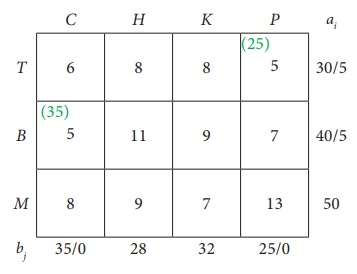Third Allocation:Fourth Allocation:Fifth Allocation:Sixth Allocation:Transportation schedule :

TŌåÆ H, TŌåÆP, BŌåÆC, BŌåÆH, MŌåÆH, MŌåÆK

The total Transportation cost = ( 5├Ś8) + (25├Ś5)+ (35├Ś5) + (5├Ś11)+ (18├Ś9) + (32├Ś7)

=  40+125+175+55+162+224

=  Ōé╣ 781

## Method:3 VogelŌĆÖs Approximation Method(VAM)

VogelŌĆÖs approximation method yields an initial basic feasible solution which is very close to the optimum solution.Various steps involved in this method are summarized as under

Step 1: Calculate the penalties for each row and each column. Here penalty means the difference between the two successive least cost in a row and in a column .

Step 2: Select the row or column with the largest penalty.

Step 3: In the selected row or column, allocate the maximum feasible quantity to the cell with the minimum cost.

Step 4: Eliminate the row or column where all the allocations are made.

Step 5: Write the reduced transportation table and repeat the steps 1 to 4.

Step 6: Repeat the procedure until all the allocations are made.

Example 10.5

Find the initial basic feasible solution for the following transportation problem by VAMSolution:

Here Ōłæai = Ōłæbj = 950

(i.e) Total Availability =Total Requirement

Ōł┤The given problem is balanced transportation problem.

Hence there exists a feasible solution to the given problem.

First let us find the difference (penalty) between the first two smallest costs in each row and column and write them in brackets against the respective rows and columnsChoose the largest difference. Here the difference is 5 which corresponds to column D1 and D2. Choose either D1 or D2 arbitrarily. Here we take the column D1 . In this column choose the least cost. Here the least cost corresponds to (S1, D1) . Allocate min (250, 200) = 200units to this Cell.

The reduced transportation table isChoose the largest difference. Here the difference is 5 which corresponds to column D2. In this column choose the least cost. Here the least cost corresponds to (S1, D2) . Allocate min(50,175) = 50 units to this Cell.

The reduced transportation table is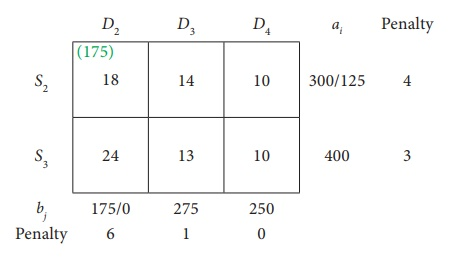Choose the largest difference. Here the difference is 6 which corresponds to column D2. In this column choose the least cost. Here the least cost corresponds to (S2, D2) .

Allocate min(300,175) = 175 units to this cell.

The reduced transportation table isChoose the largest difference. Here the difference is 4 corresponds to row S2. In this row choose the least cost. Here the least cost corresponds to (S2, D4) . Allocate min(125,250) = 125 units to this Cell.

The reduced transportation table isThe Allocation isThus we have the following allocations:Transportation schedule :

S1ŌåÆ D1, S1ŌåÆD2, S2ŌåÆD2, S2ŌåÆD4, S3ŌåÆD3, S3ŌåÆD4

This initial transportation cost

= (200 ├Ś 11) + (50 ├Ś13) + (175 ├Ś 18) + (125 ├Ś10) + (275 ├Ś13) + (125 ├Ś10)

=  Ōé╣ 12,075

Example 10.5

Obtain an initial basic feasible solution to the following transportation problem using VogelŌĆÖs approximation method.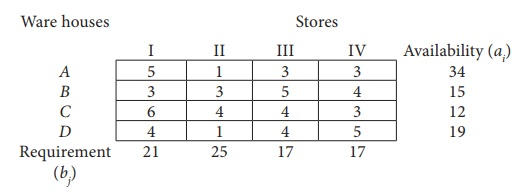Solution:

Here Ōłæai = Ōłæbj = 80 (i.e) Total Availability =Total Requirement

Ōł┤The given problem is balanced transportation problem.

Hence there exists a feasible solution to the given problem.

First Allocation:Second Allocation:Third Allocation:Fourth Allocation:Fifth Allocation:Sixth Allocation:Thus we have the following allocations:Transportation schedule :

AŌåÆ I, AŌåÆII, AŌåÆIII, AŌåÆIV, BŌåÆI, CŌåÆIV, DŌåÆII

Total transportation cost:

= ( 6 ├Ś 5)+ ( 6 + 1)+ (17 ├Ś 3)+ ( 5 ├Ś 3)+ (15 ├Ś 3) + (12 ├Ś 3)+ (1 9 ├Ś1)

= 30 + 6 + 51 + 15 + 45 + 36 + 19

= Ōé╣ 202

Tags : Solved Example Problems | Transportation Problem | Operations Research , 12th Business Maths and Statistics : Chapter 10 : Operations Research
Study Material, Lecturing Notes, Assignment, Reference, Wiki description explanation, brief detail
12th Business Maths and Statistics : Chapter 10 : Operations Research : Methods of finding initial Basic Feasible Solutions | Solved Example Problems | Transportation Problem | Operations Research